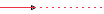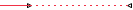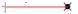Tip: If you find that the report window is too long, select Tabbed Report from the red triangle menu.
 • Event Plot
 • Compare Distributions
 • Life Distribution: Statistics
 • Life Distribution Report Options
Example of the Life Distribution Report for Fan.jmp
 • The time period when the units in the row are known to be functioning is indicated with a solid line.
 • The time period when it is not known if the units in the row are functioning is indicated with a dashed line.
 • The line terminates once the units in the row are known to have failed.
In the Fan.jmp sample data table there is a single Time column indicating failure time. When the failure time is unknown, the value Censored is recorded in the Censor column. All censored units are assumed to be right-censored. Event Plot for Right-Censored Data shows the Event Plot for this data.
Note: To construct the plot in Event Plot for Right-Censored Data, select Help > Sample Data Library and open Reliability/Fan.jmp. Select Run Script from the red triangle menu for the script Life Distribution - Exponential. Click the Event Plot disclosure icon.
Event Plot for Right-Censored Data
The unit in row 3 failed at Time 1150. Its lifetime is represented by a solid horizontal line that ends at Time 1150. The failure time is marked with an “x”.
The unit in row 5 is right censored. It was last know to be functioning at Time 1560. The time period during which the unit is known to be functioning is represented by a solid horizontal line that ends at Time 1560. At Time 1560, a right arrow is plotted. The line continues as a dashed line, indicating that the failure time is unknown, but greater than 1560.
In the Censor Labels.jmp sample data table, there are two columns, Start Time and End Time. Start Time indicates when units in a row were last known to be functioning. End Time indicates when units in that row were first known to have failed.
The Start Time and End Time values indicate the following about the units:
 • Units in rows 1 and 2 are left censored. They were known to fail before the time in the End Time column, but their exact failure times are unknown.
 • Units in rows 3 and 4 are right censored. They were known to be last functioning at the time in the Start Time column, but their failure times are unknown.
 •
 • Units in rows 7 and 8 are not censored. Their failure times are given by the values in the Start Time and End Time columns, which are identical.
Event Plot for Mixed-Censored Data shows the Event Plot for this data.
Note: To construct the plot in Event Plot for Mixed-Censored Data, select Help > Sample Data Library and open Censor Labels.jmp. Select Run Script from the red triangle menu for the script Life Distribution. Click the Event Plot disclosure icon.
Event Plot for Mixed-Censored Data
 • The patternindicates right censoring. The unit failed after its last inspection.
 • The patternindicates that the unit failed during the time interval marked by the two arrow heads.
 • The patternindicates no censoring. The unit failed at the time marked by the x.
 • the estimated cumulative distribution curve, which appears on the probability plot
 • a shaded region that indicates confidence intervals for the cumulative distribution
 • a Distribution Profiler that shows the cumulative probability of failure for a given period of time
Compare Distributions Report and Distribution Profiler shows an example of the Compare Distributions report. The Logistic (yellow) and Exponential (magenta) distributions are shown. The plot is scaled using the Exponential distribution.
Compare Distributions Report and Distribution Profiler
The available distributions are listed and described in detail in Parametric Distributions. There are four major groupings of parametric distributions:
 • Basic Failure-Time Distributions
 • Threshold Distributions
 • Defective Subpopulation Distributions
 • Zero-Inflated Distributions
You can restrict which distributions are available by default by deselecting the ones that you do not want to appear. Select File > Preferences > Platforms > Life Distribution. The distributions listed include the Threshold, Defective Subpopulation, Zero-Inflated, LogGenGamma, and GenGamma distributions. By default, all of the distributions are checked and available.
 • Lognormal
 • Weibull
 • Loglogistic
 • Fréchet
 • Normal
 • SEV
 • Logistic
 • LEV
 • Exponential
 • LogGenGamma
 • GenGamma
 • TH Lognormal
 • TH Weibull
 • TH Loglogistic
 • TH Fréchet
 • DS Lognormal
 • DS Weibull
 • DS Loglogistic
 • DS Fréchet
 • Zero-Inflated Lognormal (ZI Lognormal)
 • Zero-Inflated Weibull (ZI Weibull)
 • Zero-Inflated Loglogistic (ZI Loglogistic)
 • Zero-Inflated Fréchet (ZI Fréchet)
In the case of zero-failure data, none of the above distributions are available by default. However, you can uncheck the preference called Weibayes Only for Zero Failure Data. This enables you to obtain Bayesian fits for those distributions where the Bayesian Estimate option is available. See Weibayes Only for Zero Failure Data.
 • Lognormal
 • Weibull
 • Loglogistic
 • Fréchet
 • Normal
 • SEV
 • Logistic
 • LEV
 • Model Comparisons
 • Summary of Data
 • Nonparametric Estimate
 • Parametric Estimate - (one report appears for each distribution that you select in the Compare Distributions report)
Initially, the rows are sorted by AICc. To change the statistic used to sort the report, select Comparison Criterion from the Life Distribution red triangle menu. See Life Distribution Report Options for details about this option.
 • Covariance Matrix
 • Profilers
 • The Distribution Profiler shows cumulative failure probability as a function of time.
 • The Quantile Profiler shows failure time as a function of cumulative probability.
 • The Hazard Profiler shows the hazard rate as a function of time.
 • The Density Profiler shows the density function for the distribution.
Opens a report where you can specify the value of parameters. Enter your fixed parameter values, select the appropriate check box, and then click Update. JMP re-estimates the other parameters, covariances, and profilers based on the new parameters, and shows them in the Fix Parameter report. For an example in a competing cause situation, see Specify a Fixed Parameter Model as a Distribution for a Cause.
For the Weibull distribution, the Fix Parameter option lets you select the Weibayes method. For an example, see Weibayes Estimates. The Weibayes option is not available for interval-censored data.
Provides a calculator that enables you to estimate the mean remaining life of a unit. In the Mean Remaining Life Calculator, enter a Time and press Enter to see the estimate. Click the plus sign to enter additional times. This calculator is available for the following distributions: Lognormal, Weibull, Loglogistic, Fréchet, Normal, SEV, Logistic, LEV, and Exponential.
From the Parametric Estimate - <Distribution Name> report outline, select Bayesian Estimates. This opens an outline called Bayesian Estimation - <Distribution Name>. The initial report is a control panel where you can specify the parameters for the priors and control aspects of the simulation.
 •
 •
 •
 a. Specify failure probability by estimates and error percentages. The prior information for each Beta prior distribution can be specified using a probability estimate and an estimate error. See Kaminskiy and Krivtsov, 2005.
 b. Specify failure probability estimate ranges. You can specify the 99% range for the two Beta distributions in the following ways:
 ‒ For each failure time, enter an initial value for the Lower and Upper 99% Limits.
 ‒ Click on the vertical line segments in the graph and drag them to your two time points. Adjust the vertical spread of each marker to specify the 99% limits.
 ‒
 ‒ The predicted value is the median of these calculated values.
 ‒ The upper and lower confidence limits are the 0.025 and 0.975 quantiles of these calculated values.
The plot and confidence limits shown in the Quantile Profiler are obtained in a similar fashion. For a given Probability value p, the quantiles corresponding to p are calculated from the distributions associated with the posterior parameter values.
Note: By default, zero-failure data is analyzed using the Weibayes method. If you want to conduct a broader Bayesian analysis on zero-failure data, uncheck the preference Weibayes Only for Zero Failure Data, located under File > Preferences > Platforms > Life Distribution.
In the Estimate Probability calculator, enter a value for Time. Press Enter to see the estimates of failure probabilities, survival probabilities, and corresponding confidence intervals. To calculate multiple probability estimates, click the plus sign, enter another Time value in the box, and press Enter. Click the minus sign to remove the last entry.
In the Estimate Quantile report, enter a value for Failure Probability. Press Enter to see the quantile estimates and corresponding confidence intervals. To calculate multiple quantile estimates, click the plus sign, enter another Failure Probability value in the box, and press Enter. Click the minus sign to remove the last entry.